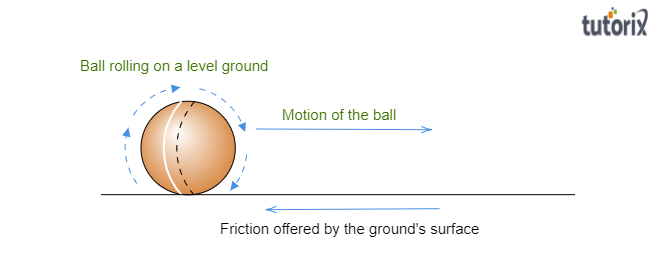# A batsman hits a cricket ball which then rolls on level ground.After covering a short distance, the ball comes to rest. The ball slows to a stop because$(a)$. the batsman did not hit the ball hard enough.$(b)$. velocity is proportional to the force exerted on the ball.$(c)$. there is a force on the ball opposing the motion.$(d)$. there is no unbalanced force on the ball, so the ball would want to come to rest.

The correct answer is: $(c)$. there is a force on the ball opposing the motion.

Explanation:

It is given that when a batsman hits a cricket ball, then rolls on level ground. After covering a short distance, the ball comes to rest. We have to find a possible reason why the rolling ball came to rest after traveling a short distance. Let us find the possible reason:The reason for coming to the ball into rest:

The ball comes to rest due to forces $(e.g.\ friction\ and\ air\ resistance)$ opposing the motion of the ball. When the ball rolls over the surface of the ground, there is a friction force acting between the surfaces of the ground and the ball, and there is also air resistance that opposes the motion of the ball. Thus the ball stops due to these opposing forces. Let us know a little about friction:

Friction:

When an object moves on the surface of another object, there is a force that opposes this motion acting between the surfaces of two objects, it is known as friction force.